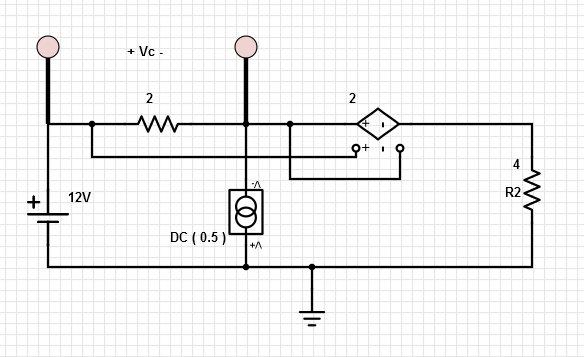# Power and Dependent Sources

Determine the power absorbed by the VCVS in the figure. Solution: The VCVS consists of an open circuit and a controlled-voltage source. There is no current in the open circuit,so no power is absorbed by the open circuit. The voltage vc across the open circuit is the controlling signal of the VCVS. The voltage Vc (across 2 ohm resisitor) measures vc to be vc = 2V. The voltage of the controlled voltage source is vd = 2 vc = 4V. The current in the controlled voltage source is 6V/4 ohm= 1.5A. The element current id and voltage vd adhere to the passive convention. Therefore, p = id*vd = (1.5)(4) = 6W is the power absorbed by the VCVS.

For more detail: Power and Dependent Sources